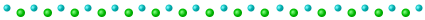# advent of code 2021 in J

2021-12-02

word count: 549

this year i decided to solve advent of code in both racket and j. you can check my solutions for both here. i won't talk about racket cause it's a more "normal" language, but i want to explain my solutions for j cause i think they are fun and cool

note: you can read both parts for every problem here, i'm keeping a mirror so you don't have to have an account to read the problems

you shouldn't need much previous j knowledge to be able to read this, but you should know that:

• j is interpreted right to left, so `2 * 3 + 1` is equal to `8`

• assignments are done with either `=.` or `=:`

• comments are started with `NB.`

• verbs is what j calls functions, and adverbs are things that modify verbs

this isn't really an intro to the language, so you might not understand some stuff if you've never used an array language before. i want to write an actual intro to j some day, i'll link it here whenever i get around to it

## day 1

this problem asks us to count how many increases there are in a list of numbers. this is very suited for array languages like j, as you can see by the concise-ness of the solution

```  NB. open file and interpret as numbers
in =. ". 'm' fread '../input/day1.txt'

NB. part 1
+/ 2 </\ in

NB. part 2
+/ 2 </\ 3 +/\ in
```

in this problem we first use `'m' fread '../input/day1.txt'` to read the file into an array of lines, and by using `".`, we interpret the strings as numbers.

after that, we do `2 </\ in`. here we come into contact into some of the weirdness of j. `</\` is a verb formed by the verb `<`, and the adverbs `/` and `\`. `<` is the less than verb, which we combine with `/`, commonly called insert, which inserts the verb between all the elements of the array: `</ 1 2 3` is the same as `1 < 2 < 3`. this is combined with the `\` adverb (called infix), which creates a rolling window and then applies the verb. the `2` in `2 </\ in` is how long the window should be

with this, we have an array with 1 where there's an increase. we just need to add them all up using `+/` to get the total number of increases there are

the second part is pretty much the same, but before doing `2 </\`, we first do a sum of a rolling window of length 3

## day 2

this problem involves submarines, so you already know it's cool :)

we're given a list of instructions that guide the submarine, and we have to interpret them to know what the final destination of it is

to solve this problem we abuse `".`, which is the eval verb, usually called do

```  NB. open file

NB. part 1
NB. (depth, horizontal)
up =: (-1, 0)&*
down =: (1, 0)&*
forward =: (0, 1)&*

*/ +/ ". in

NB. part 2
aim =. 0

up =. {{ (0, 0) [aim =: aim - y }}
down =. {{ (0, 0) [aim =: aim + y }}
forward =. {{ (y * aim), y }}

*/ +/ ". in
```

we define a verb for each of the three commands, and we make them multiply the input they get with different arrays, like `(-1, 0)`. then, by doing `". in"`, we get an array of arrays, where for each element, the first element is the depth, and the second is the horizontal coordinate. with that, we can use `+/` to add all of the depths and all of the horizontals to get a two element array, which we can then multiply with `*/` to get our final result

the second part is similar, but it also has an `aim` variable, which we modify in the `up` and `down` verbs. the final expression is the same as in part 1